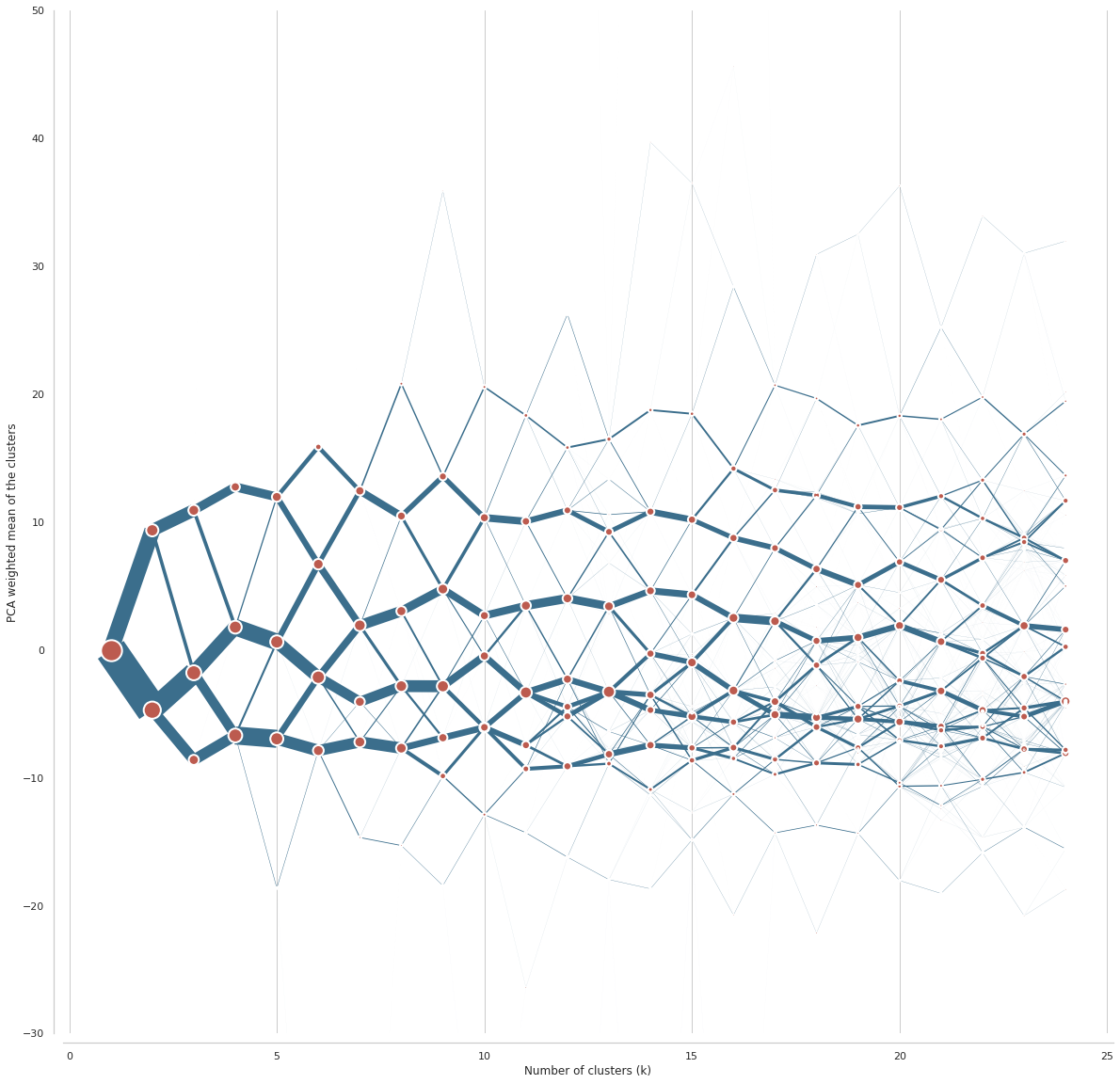# Generate Spatial Signatures across GB¶

This notebook generates spatial signatures as a clustering of form and function characters.

## Number of clusters¶

Clustergram which will be used to determine number of clusters will likely be complex. It is better to use new interactive version (`clustergram=>0.5.0` required).

```# pip install git+https://github.com/martinfleis/clustergram.git
```
```import dask.dataframe
import numpy as np

from clustergram import Clustergram
```

We first load all standardized data and create a single pandas DataFrame.

```standardized_form = dask.dataframe.read_parquet("../../urbangrammar_samba/spatial_signatures/clustering_data/form/standardized/").set_index('hindex')
data = dask.dataframe.multi.concat([standardized_form, stand_fn], axis=1).replace([np.inf, -np.inf], np.nan).fillna(0)
```
```%time data = data.compute()
```
```CPU times: user 2min 36s, sys: 1min 24s, total: 4min
Wall time: 2min 43s
```

Due to a small mistake, parquet files contain 3 columns which were used temporarily during the computation. We remove them now.

```data = data.drop(columns=["keep_q1", "keep_q2", "keep_q3"])
```

We run clustergram for all the options between 1 and 24 clusters using Mini-Batch K-Means algorithm.

```cgram = Clustergram(range(1, 25), method='minibatchkmeans', batch_size=1_000_000, n_init=100, random_state=42)
cgram.fit(data)
```
```K=1 fitted in 780.0785899162292 seconds.
K=2 fitted in 864.1424376964569 seconds.
K=3 fitted in 954.2592947483063 seconds.
K=4 fitted in 1258.9098596572876 seconds.
K=5 fitted in 1360.8928196430206 seconds.
K=6 fitted in 1446.0337007045746 seconds.
K=7 fitted in 1550.0224254131317 seconds.
K=8 fitted in 1662.5290818214417 seconds.
K=9 fitted in 1759.5144119262695 seconds.
K=10 fitted in 1860.4208154678345 seconds.
K=11 fitted in 1957.2675037384033 seconds.
K=12 fitted in 2036.3741669654846 seconds.
K=13 fitted in 2098.1098449230194 seconds.
K=14 fitted in 2188.7303895950317 seconds.
K=15 fitted in 2251.541695833206 seconds.
K=16 fitted in 2390.264476776123 seconds.
K=17 fitted in 2506.9812376499176 seconds.
K=18 fitted in 2602.7613401412964 seconds.
K=19 fitted in 2642.102708339691 seconds.
K=20 fitted in 2746.4516792297363 seconds.
K=21 fitted in 2901.3386924266815 seconds.
K=22 fitted in 3010.796851873398 seconds.
K=23 fitted in 3055.145115852356 seconds.
K=24 fitted in 3155.7938318252563 seconds.
```

We can save resulting labels to parquet.

```labels = cgram.labels.copy()
labels.columns = labels.columns.astype("str")  # parquet require str column names
labels.to_parquet("../../urbangrammar_samba/spatial_signatures/clustering_data/clustergram_labels.pq")
```

Now we can plot the clustergram.

```import urbangrammar_graphics as ugg
import seaborn as sns

sns.set(style='whitegrid')
```
```%%time

ax = cgram.plot(
figsize=(20, 20),
line_style=dict(color=ugg.COLORS),
cluster_style={"color": ugg.COLORS},
)
ax.yaxis.grid(False)
sns.despine(offset=10)
ax.set_ylim(-30, 50)
```
```CPU times: user 11min 28s, sys: 4min 51s, total: 16min 20s
Wall time: 3min 30s
```
```(-30.0, 50.0)
```Better option is an interactive clustergram, showing the same data in a more friendly manner. We first initialise bokeh.

```from bokeh.io import output_notebook
from bokeh.plotting import show

output_notebook()
```

Now we can plot clustergram using bokeh. First the same as above, using PCA weighting.

```fig = cgram.bokeh(
figsize=(800, 600),
line_style=dict(color=ugg.HEX),
cluster_style={"color": ugg.HEX},
)
show(fig)
```

Second, we can plot clustergram using mean values. Both perspectives combined give us a better picture of clustering behaviour.

```fig2 = cgram.bokeh(
figsize=(800, 600),
line_style=dict(color=ugg.HEX),
cluster_style={"color": ugg.HEX},
pca_weighted=False
)
show(fig2)
```